• Graph_AutoEncoder_with_GCMC Graph_AutoEncoder_with_GCMCPython
• <p>fix gcmc segfaults with mol keyword <h2>Type of Issue <p>Bug Report <h2>LAMMPS Version (Bug Report) <p>I have two installs of lammps. Gentoo default install (9Jan2017) and my own manual install for...
• <div><p>I have tried to do GCMC of water molecules with ReaxFF KOKKOS (11 april). Every time <code>run</code> is called with an active <code>fix gcmc, the <code>init</code> function is called, which ...
• m running a GCMC calculation with ReaxFF KOKKOS (11 april). The attached script works on CPU, but not on GPU (any number). I have tried with NVidia GTX Titan and NVidia P100. <p>The callstack is: ...
• ) neighbor list and gcmc. In the attached example, I try to insert oxygen atoms into a system using the vashishta potential. <p>This works with for instance LAMMPS (27 Oct 2016-ICMS), but the current...
• For dynamics this is usually not happening due to repulsion, but <code>fix gcmc</code> might either insert or translate atoms past the repulsion barrier. This results in an physically invalid ...
• 谈谈LAMMPS中的GCMC（巨正则蒙特卡洛） 记录学习心得（2020.2.21） 疫情期间，还是在家专心科研，养成好习惯，继续记录。 Example ：fix ID group-ID gcmc N X M type seed T mu displace keyword values N：每N步...
谈谈LAMMPS中的GCMC（巨正则蒙特卡洛）
Example ：fix ID group-ID gcmc N X M type seed T mu displace keyword values
N：每N步进行一次MC操作，每次操作进行 X 次的交换和 M 次的移动和旋转；
type： 原子type；
Seed：随机数种子；
T：温度；
Mu：化学势；
Displace：最大平移距离；（提前设置一个量，防止移动太远）
Keyword : {:分子模板 ;:MC移动的区域 ;:分子最大旋转角度 ;: 气体压力（与体系耦合的大气体浴）; <full_energy>:Metropolis比较的时候，计算full energy判断 (复杂体系);<intra_energy>:需要交掉的分子内势能（rigid or shake, …dont worry…） ; :新加入分子的group ; <overlap_cutoff>: overlap拒接受的最大距离}。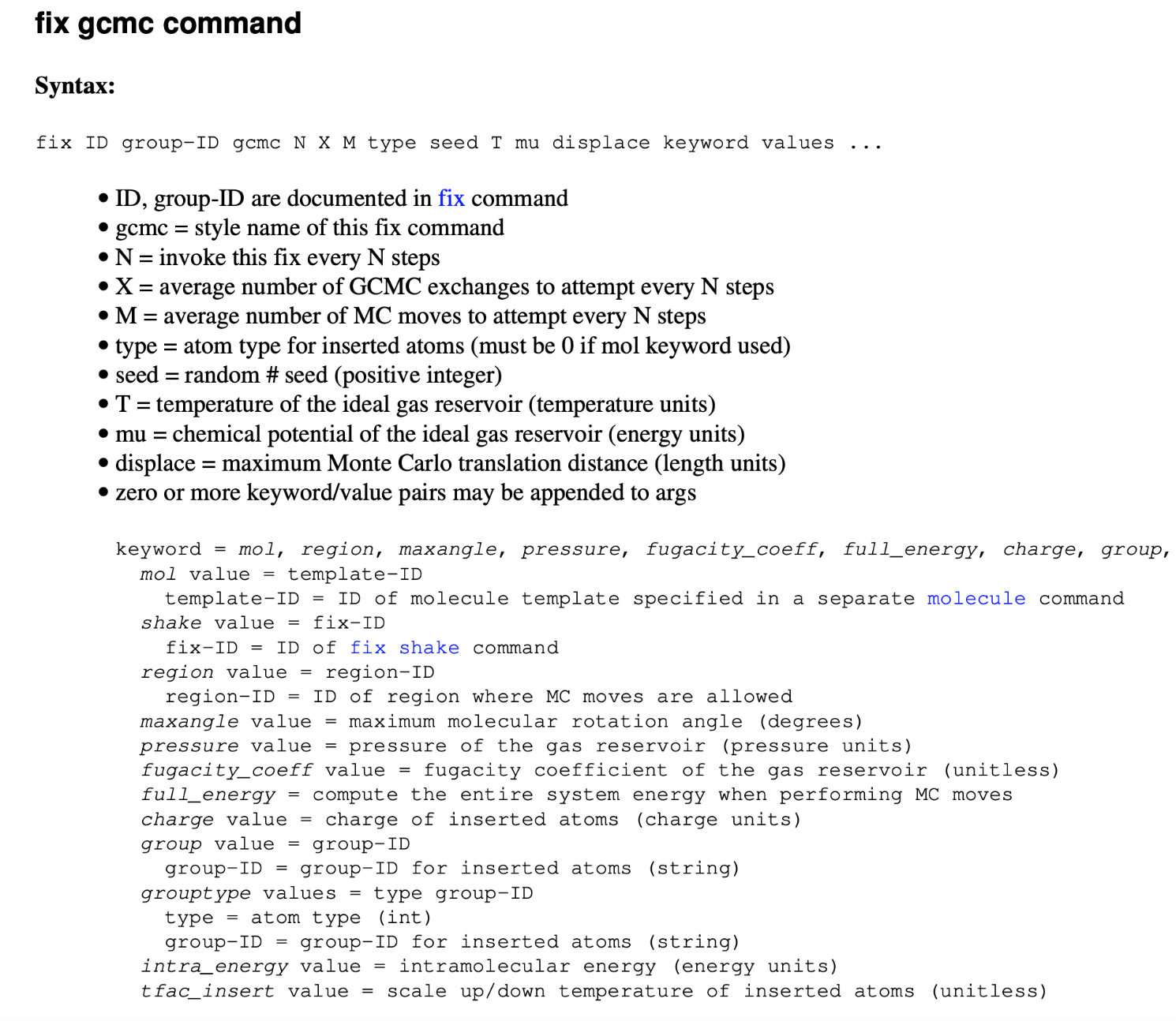上面是简单的命令介绍，打开LAMMPS的EXAMPLE看看里面有几个case可以学习。
# GCMC for LJ simple fluid, no dynamics
# T = 2.0
# rho ~ 0.5
# p ~ 1.5
# mu_ex ~ 0.0
# comparable to Frenkel and Smit GCMC Case Study, Figure 5.8

# variables modifiable using -var command line switch

variable        mu index -1.25
variable        temp index 2.0
variable	disp index 1.0
variable        lbox index 5.0

# global model settings

units           lj
atom_style      atomic
pair_style      lj/cut 3.0
pair_modify	tail no # turn of to avoid triggering full_energy

# box

region		box block 0 ${lbox} 0${lbox} 0 ${lbox} create_box 1 box # lj parameters pair_coeff * * 1.0 1.0 mass * 1.0 # we recommend setting up a dedicated group for gcmc group gcmcgroup type 1 # gcmc fix mygcmc gcmcgroup gcmc 1 100 100 1 29494${temp} ${mu}${disp}

# atom count

variable 	    type1 atom "type==1"
group 		     type1 dynamic gcmcgroup var type1  ## 设置动态group 粒子数可以变化
variable        n1 equal count(type1)   ###统计粒子数

# averaging

variable	   rho equal density
variable	   p equal press
variable	   nugget equal 1.0e-8
variable      lambda equal 1.0
variable     	muex equal ${mu}-${temp}*ln(density*${lambda}+${nugget})
fix 		        ave all ave/time 10 100 1000 v_rho v_p v_muex v_n1 ave one file rho_vs_p.dat
variable	    rhoav equal f_ave
variable	    pav equal f_ave
variable	    muexav equal f_ave
variable	    n1av equal f_ave

# output

variable	tacc equal f_mygcmc/(f_mygcmc+${nugget}) variable iacc equal f_mygcmc/(f_mygcmc+${nugget})
variable	dacc equal f_mygcmc/(f_mygcmc+${nugget}) ### particle number is not a contant compute_modify thermo_temp dynamic yes thermo_style custom step temp press pe ke density atoms v_iacc v_dacc v_tacc v_rhoav v_pav v_muexav v_n1av thermo 1000 run 10000   展开全文lammps • m working on water loading inside zeolites with ReaxFF and fix_gcmc using the KOKKOS package (<code>kokkos_omp, 1 MPI process, 32 threads) with LAMMPS (11 Apr 2017). I've used the <code>intra_... • <p>Adds the tail corrections to the <code>fix gcmc</code> during insertions and deletions <h2>Author(s) <p>Marshall McDonnell (Oak Ridge National Laboratory) <h2>Backward Compatibility <p>This will ... • <p>As been reported in #467, and also on the mailing list, LAMMPS is broken with <code>fix gcmc</code> using molecule on more than one MPI process. With this PR, LAMMPS detects this and throws an ... • m working with GCMC with water molecules in zeolites using ReaxFF. I've had problems where atoms suddenly are moved closer than what is allowed by the overlap_cutoff. I think the problem is ... • 今天学习的是 KDD18 的一篇...这篇论文在 VGAE 的基础上提出了 GCMC 模型，设计了一个可微的基于消息传递的图自编码框架进行矩阵补全（matrix completion），同时考虑边信息和网络结构，并分析了边信息在推荐系统冷启动 今天学习的是 KDD18 的一篇论文《Graph Convolutional Matrix Completion》，作者是阿姆斯特大学的同学，Thomas N. Kipf 大佬是二作。 前面我们介绍了 Kipf 大佬利用变分自编码器（VGAE）来完成链接预测问题，链接预测问题放在矩阵中可以被认为是矩阵补全。这篇论文在 VGAE 的基础上提出了 GCMC 模型，设计了一个可微的基于消息传递的图自编码框架进行矩阵补全（matrix completion），同时考虑边信息和网络结构，并分析了边信息在推荐系统冷启动的影响。 1.Introduction 先简单介绍下二部图（bipartite graph）。 二部图是一种特殊的图结构，所有的顶点可以被分割为两个互不相交的子集（U，V），并且每条边 $e_{ij}$ 所关联的顶点 $i,j$ 分别属于这两个不同的顶点集合 $(i\in U,j\in V)$。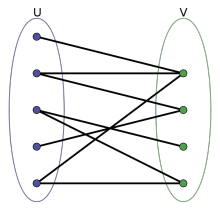二部图的应用非常广泛，比如说电影推荐这样的交互数据则可以用一个二部图来表示（user-movie），图的边则是用户对电影的评分，此时的矩阵补全就是预测用户的观看后的评分。 作者在这篇论文中提出的 GCMC 框架是一种对矩阵进行补全的图自编码框架，其利用 user 和 item 的交互信息生成 user 和 item 之间的隐特征，并通过双线性解码器来重建 user 和 item 之间的链接。 这篇论文的主要贡献主要有两点： 将 GNN 应用于带有 side information 的矩阵补全任务中，并证明基于消息传递的模型比之前的复杂模型具有更好的性能； 引入了 Dropout 正则化技术：以一定概率删除单个节点的所有传出消息。 2.GCMC 下图为 GCMC 的基本流程，在二部图的矩阵补全任务转换成比链接预测问题，并使用端到端的图自编码器进行建模：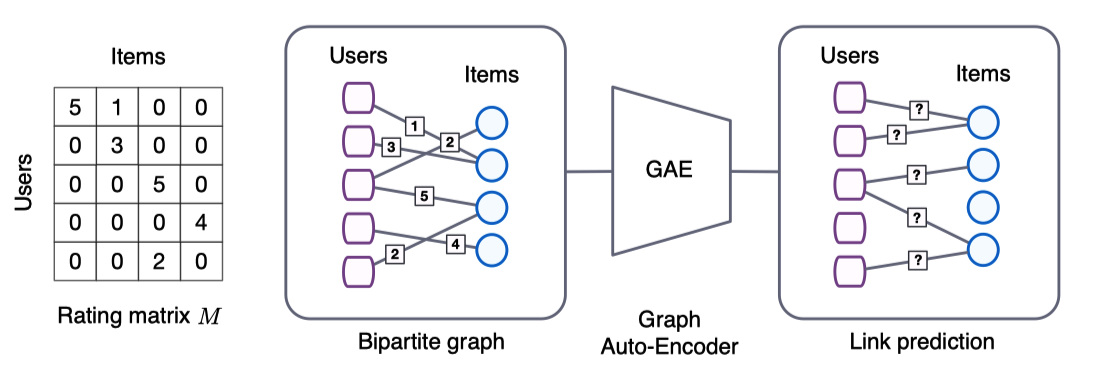2.1 Graph convolutional encoder 首先来看编码器。 本文针对推荐任务提出了图卷积编码器，其能够有效的利用卷积操作的权值共享。图数据的局部卷积操作只考虑节点的直接邻居，因此可以应用于图数据中的所有位置。 我们也知道，局部图卷积可以看作是一种消息传递，节点的特征值沿着边进行传递和转换。作者设计了一种基于评分等级的转换，从 item j 到 user i 的信息传递被定义为： $\mu_{j\rightarrow i,r} = \frac{1}{c_{ij}} W_r x_j^v \\$ 其中，$c_{ij}$ 为正则化常数，可以为左正则化 $|N(u_i|$ 也可以为对称正则化 $\sqrt{|N(u_i)||N(u_j)|}$。$N(u_i)$ 表示 user i 的邻居集合；$W_r$ 为基于边类型（评分等级）的参数矩阵；$x_j^v$ 表示 item j 的特征向量。 从 user 到 item 的消息传递也可以采用类似的方式，这个过程称为图卷积层。 在消息传递完成之后，每一个节点都会对消息进行累积操作： $h_{i}^{u}=\sigma\left[\operatorname{accum}\left(\sum_{j \in \mathcal{N}_{i}\left(u_{i}\right)} \mu_{j \rightarrow i, 1}, \ldots, \sum_{j \in \mathcal{N}_{R}\left(u_{i}\right)} \mu_{j \rightarrow i, R}\right)\right] \\$ 其中，$\operatorname{accum}(\cdot)$ 为聚合运算，如 stack、sum 等；$\sigma(\cdot)$ 为激活函数。 对 $h_i^u$ 进行转换便能得到 user 的 Embedding： $z_i^u = \sigma(Wh_i^u) \\$ 其中，W 为参数矩阵。 计算 item 的 Embedding $z_i^v$ 方法类似，并共享参数矩阵，这个过程称为稠密层。 在实验过程中，堆叠图卷积层并不能提高效果，但是在卷积层后连一个稠密层效果会好很多。 2.2 Bilinear decoder 再来看下解码器。 作者提出了一个双线性解码器（bilinear decode），把用户对物品的评分等级视为多类。$\check M$ 表示为 user 和 item 之间重构的评分矩阵。解码器可以通过对可能的评分等级进行双线性运算，然后用 softmax 函数生成一个概率分布： $p\left(\check{M}_{i j}=r\right)=\frac{e^{\left(z_{i}^{u}\right)^{T} Q_{r} z_{j}^{v}}}{\sum_{s=1}^{R} e^{\left(z_{i}^{u}\right)^{T} Q_{s} z^v_{j}}} \\$ 其中，$Q_r \in \mathbb{R}^{H\times H}$ 是一个可训练的参数共享矩阵（后面进行介绍），H 为节点的隐特征的维度。 预测的评分等级的计算方式为： $\check{M}_{i j}=g\left(u_{i}, v_{j}\right)=\mathbb{E}_{p\left(\check{M}_{i j}=r\right)}[r]=\sum_{r \in R} r\; p\left(\check{M}_{i j}=r\right) \\$ 2.3 Model train 然后看一下模型训练。 考虑 Loss Function 使用交叉熵损失函数： $\mathcal{L}=-\sum_{i, j ; \Omega_{i j}=1} \sum_{r=1}^{R} I\left[M_{i j}=r\right] \log p\left(\check{M}_{i j}=r\right) \\$ 其中，$I[k=l]$ 为指示函数，$k=l$ 是为 1，否则为 0；$\Omega\in{0,1}^{N_u\times N_v} \$ 用来表示链接是否存在，其作用类似 mask；
考虑 Mini-batch

对于不同评分类别将进行固定数量的 pair-wise 采样，一来减少训练所需内存，二来达到了正则化的效果；
通过通过实验验证了 mini-batch 和 full-batch 的效果类似；
在大数据集中应用了 mini-batch，而在小数据中应用 full-batch 以获得更快的收敛速度。

考虑 Node Dropout

为了泛化模型，作者在训练过程中将以一定概率随机删除节点的所有传出信息；
实验表明，节点 Dropout 比消息的 Dropout 更有效。

考虑 weight sharing

考虑 user 对 item 打分的非均匀性，防止权重矩阵 $W_r$ 的列优化不均匀，作者使用了一种在不同评分关系之间进行参数共享的方法：

$W_r = \sum_{s=1}^r T_s \\$
其中，$T_s$ 为基础矩阵，评分越高，$W_r$ 包含的 $T_s$ 数量越多。作者称之为序数权值共享（ordinal weight sharing）。
解码器中的权重矩阵 $Q_r$ 就是采用一组基于基础参数矩阵的线形组合的参数共享矩阵：
$Q_{r}=\sum_{s=1}^{n_{b}} a_{r s} P_{s} \\$
其中，$n_b$ 表示基权重矩阵 $P_s$ 的数量；$a_{rs}$ 为可学习的系数。
为避免过拟合，同时减少参数的数量，基权重矩阵的数量要小于评分级别的数量。
2.4 Feature representation and side information
考虑建模时的除了节点表征外的辅助信息（side info），以 user 节点为例：
$z_{i}^{u}=\sigma\left(W h_{i}^{u}+W_{2}^{u, f} f_{i}^{u}\right) \quad \text { with } \quad f_{i}^{u}=\sigma\left(W_{1}^{u, f} x_{i}^{u, f}+b^{u}\right) \\$
其中，$W_1^{u,f}$ 和 $W_2^{u,f}$ 为可训练的权重矩阵；$b^u$ 为一个 bias，$x_i^{u,f}$ 为节点 i 的辅助信息。
item 节点同理，把 u 改成 v 即可。
3.Experiments
简单看一下实验。
先看下数据集：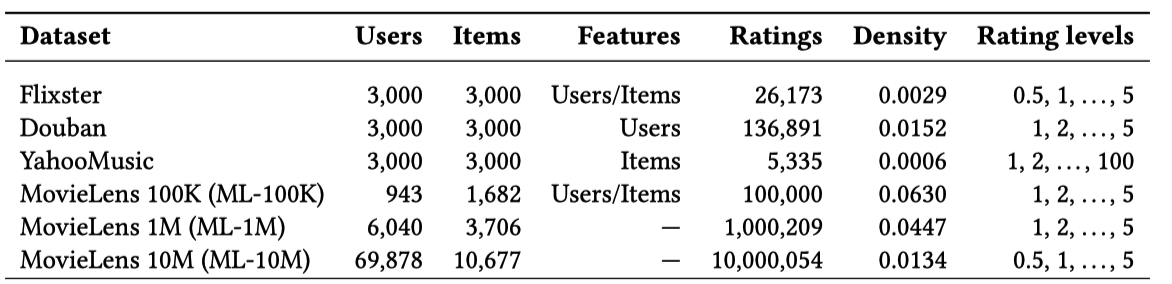然后是不同模型在 ML-100K 上的表现，评分标准为 RMSE：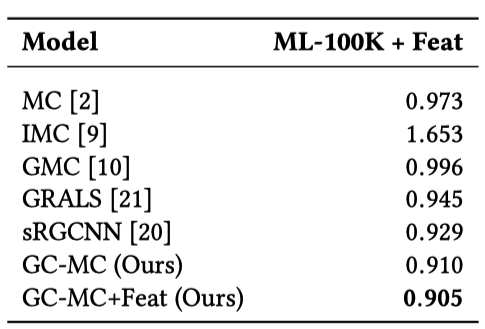数据集大一点的情况下：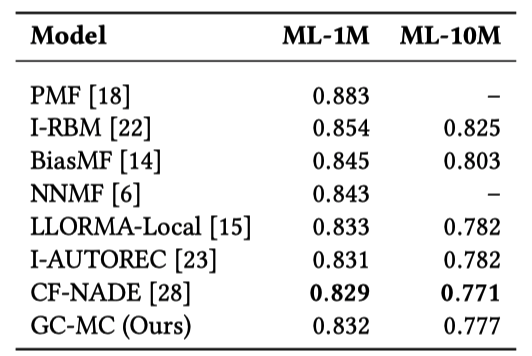效果不满意再试一下其他数据集：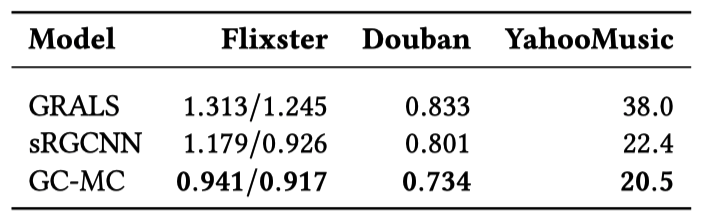效果满意了，我们再分析下冷启动：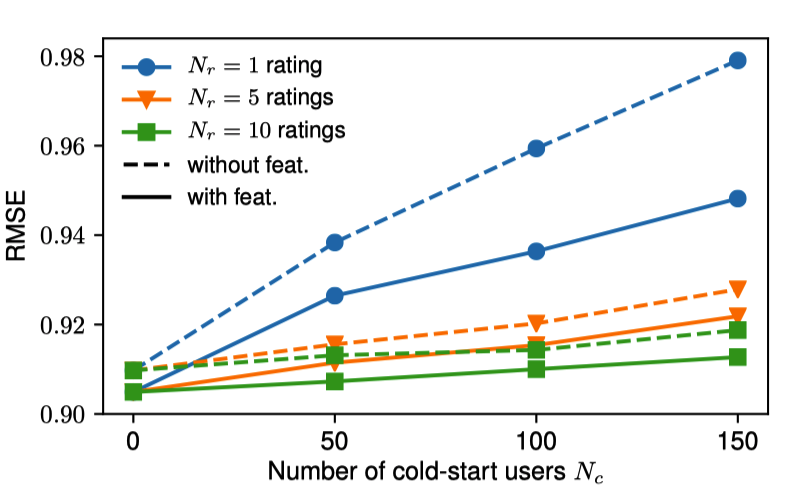4.Conclusion
总结：GCMC 利用基于消息传递的图卷积网络将节点特征编码为隐特征，并利用双线形解码器预测未知边的不同评分值的概率评分，并取其期望作为边的预测评分。
5.Reference

Berg R, Kipf T N, Welling M. Graph convolutional matrix completion[J]. arXiv preprint arXiv:1706.02263, 2017.
Github：gc-mc

关注公众号跟踪最新内容：阿泽的学习笔记。展开全文机器学习 人工智能 深度学习 图神经网络
• 图卷积矩阵完成（Pytorch） 重新实现（PyTorch和PyTorch Geometric） 笔记 该存储库不是本文的正式实现。 官方的实现是（Tensorflow）。 我们的实验结果如下所示，但未达到原始分数。 设置 使用python 3.6或更高...Python
• 据此，作者提出了一个图卷积矩阵补全（GCMC）框架：在用深度学习处理图结构的数据的研究进展的基础上，对矩阵进行补全的一种图自编码器框架。这个自编码器通过在二部交互图中信息传递的形式生成user和item之间的隐含...
文章目录1 相关介绍1.1 背景1.2 side information1.3 contributions1.4 相关介绍自编码器矩阵分解模型Matrix completion with side information2 在二部图中矩阵补全作为一种连接预测2.1 符号定义2.2 Revisiting graph auto-encoders 图自编码器2.3 Graph convolutional encoder 图卷积编码器2.4 Bilinear decoder 双线性解码器2.5 模型训练Loss functionMini-batchingNode dropoutWeight sharing2.6 Input feature representation and side information3 实验3.1 MovieLens 100K3.2 MovieLens 1M and 10M3.3 Flixster, Douban and YahooMusic3.4 Cold-start analysis3.5 讨论4 总结
论文：Graph Convolutional Matrix Completion （GCMC） 图卷积矩阵补全
作者：来自于荷兰阿姆斯特丹大学的Rianne van den Berg, Thomas N. Kipf（GCN的作者）, Max Welling
来源：KDD 2018 Workshop
论文链接：https://www.kdd.org/kdd2018/files/deep-learning-day/DLDay18_paper_32.pdf
Github链接：https://github.com/riannevdberg/gc-mc
图卷积神经网络（GCN）是现在深度学习的热点之一，这篇文章基于user-item的二部图（Bipartite graph），提出了一种图自编码器框架，从链路预测的角度解决推荐系统中的评分预测问题。此外，为了验证所提出的消息传递方案，在标准的协同过滤任务上测试了所提出的模型，并展示出了一个有竞争力的结果。
1 相关介绍
1.1 背景
随着电子商务和社交媒体平台的爆炸式增长，推荐算法已经成为许多企业不可或缺的工具。
其中，推荐系统的一个子任务就是矩阵补全。文中把矩阵补全视作在图上的链路预测问题：users和items的交互数据可以通过一个在user和item节点之间的二分图来表示，其中观测到的评分/购买用links来表示。因此，预测评分就相当于预测在这个user-item二分图中的links。
据此，作者提出了一个图卷积矩阵补全（GCMC）框架：在用深度学习处理图结构的数据的研究进展的基础上，对矩阵进行补全的一种图自编码器框架。这个自编码器通过在二部交互图中信息传递的形式生成user和item之间的隐含特征。这种user和item之间的隐含表示用于通过一个双线性的解码器重建评分links。
当推荐图中带有结构化的外部信息(如社交网络)时，将矩阵补全作为二部图上的链接预测任务的好处就变得尤为明显。将这些外部信息与交互数据相结合可以缓解与冷启动问题相关的性能瓶颈。实验证明了作者提出的的图自编码器模型能够有效地将交互数据与side information结合起来。进一步证明了，在纯协同过滤场景中，这种方法能够与最新最先进的方法竞争。
1.2 side information
边信息（Side Information）：是指利用已有的信息Y辅助对信息X进行编码，可以使得信息X的编码长度更短。
边信息一个通俗的例子是：假设到马场去赌马，根据每个马的赔率可以得到一个最佳的投资方案。但是如果知道赌马的一些历史数据，例如上几场的胜负情况，那么可以得出一个更优的投资方案。赌马中的历史数据就是边信息。
1.3 contributions

将图神经网络应用于带有结构化side information的矩阵补全任务，并证明这种简单消息传递模型比基于复杂图形的方法有更好的性能
我们引入了节点dropout，这是一种有效的正则化技术，它以固定的概率删除单个节点的所有传出消息的整个集合

1.4 相关介绍
自编码器
下面的基于user或item的自动编码器是一类最新最先进的协同过滤模型，可以看作是文中的图数据自编码器模型的一个特例，其中编码器只考虑user或item的embedding。

Autorec: Autoencoders meet collaborative filtering，WWW 2015
Dropout: a simple way to prevent neural networks from overfitting，2014
[CF-NADE] A neural autoregressive approach to collaborative filtering，ICML 2016

Autorec是这的第一个这样的模型，在这个模型中，其中，部分观察到的user或item的评分向量通过编码器层投影到潜在空间，并使用均方重建误差损失的解码器层重建。
CF-NADE算法可视为上述自动编码器体系结构的一个特例。在基于user的场景中，消息只从items传递给users
，在基于item的情况下，反之亦然。和文中的自编码器不同的是，评级的items在编码器中被分配了默认的3级，从而创建了一个完全连接的交互图。CF-NADE在节点上强制执行随机排序，并通过随机剪切将传入的消息分成两组，只保留其中一组。因此，该模型可以看作是一个去噪自动编码器，在每次迭代中，输入空间的一部分被随机丢弃。
矩阵分解模型
文中提出的模型和很多矩阵分解方法有关：

概率矩阵分解(Probabilistic matrix factorization，2008) (PMF)：采样概率的方法，将矩阵$M$分解为$M \approx U V^{T}$
BiasedMF( Matrix Factorization Techniques for Recommender Systems，2009):通过合并一个user和item的特定bias以及全局bias来改进PMF
神经网络矩阵分解 （Neural network matrix factorization，2015） (NNMF)：扩展了MF方法，通过前馈神经网络传递潜在的users和items特征
局部低秩矩阵近似（ Local low rank matrix approximation，ICML 2013）：利用低秩近似的不同(与entry相关)组合来重建评价矩阵entries

Matrix completion with side information

在matrix completion (MC)（Exact matrix completion via convex optimization，2012）中，目标是用一个低秩评分矩阵去近似一个评分矩阵。然而，秩最小化是一个棘手的问题，论文中将秩最小化替换为核范数最小化(矩阵奇异值的总和)，将目标函数转化为可处理的凸函数。
Inductive matrix completion (IMC)（Provable inductive matrix completion，2013）将users和items的内容信息合并到特征向量中，将评分矩阵中观察到的元素近似为$M_{i j}=x_{i}^{T} U V^{T} y_{j}$，其中$x_i$和$y_j$分别代表user$i$和item$j$的特征向量。
geometric matrix completion (GMC) model（Matrix completion on graphs，2014）通过以user图和item图的形式添加side information，引入了MC模型的正则化
Collaborative Filtering with Graph Information: Consistency and Scalable Methods（INPS 2015） 针对图正则化矩阵补全问题，提出了一种更有效的交替最小二乘优化方法(GRALS)。
RGCNN（Geometric matrix completion with recurrent multi-graph neural networks，NIPS 2017） 是和本文最相关的工作。探讨了基于切比雪夫多项式的users和items k-nearest图的谱图滤波器的应用。文中的模型在一个编码器-解码器步骤中直接建模评级图，而不是使用递归估计，速度有显著的提升。
PinSage，这是一个高度可扩展的图卷积网络，基于GraphSAGE框架，用于推荐的web级图，其中对邻居进行下采样以增强可扩展性。与PinSage相比，此文关注包含于图的side information，例如以社交网络图的形式，并进一步引入正则化技术来提高泛化。

2 在二部图中矩阵补全作为一种连接预测
2.1 符号定义

$M$:评分矩阵，维度为$N_u × N_v$，其中$N_u$是users的数量，$N_v$是items的数量
非零的$M_{ij}$表示user$i$对item$j$的评分，$M_{ij}=0$表示一个没有观测到评分图1表示了整个模型的流程。在一个二分的user-item交互图中，矩阵补全任务(即对未观察到的交互的预测)可以转换为链接预测问题，并使用端到端可训练的图自编码器进行建模。

交互数据可以用无向图G表示：$G=(\mathcal{W}, \mathcal{E}, \mathcal{R})$
$\mathcal{W}=\mathcal{W}_{u} \cup \mathcal{W}_{v}$；$\mathcal{W}_{u}$表示user节点的集合,维度为$N_u$；$\mathcal{W}_{v}$表示item节点的集合,维度为$N_v$
边 $\left(u_{i}, r, v_{j}\right) \in \mathcal{E}$带有含评分等级类型的标签，$r \in\{1, \ldots, R\}=\mathcal{R}$

2.2 Revisiting graph auto-encoders 图自编码器
先前的推荐系统的基于图的方法通常采用多级pipline（此论文有介绍：Recommendation as link prediction in bipartite graphs: A graph kernel-based machine learning approach），其中包括图特征提取模型和链接预测模型，所有这些都分别进行训练。 然而，通过使用端到端学习技术对图结构数据进行建模，通常可以显着改善结果，特别是使用图自动编码器用于在无向图上进行无监督学习和链接预测。
本文采用（Thomas N. Kipf and Max Welling. Variational graph auto-encoders. NIPS Bayesian Deep Learning Work- shop, 2016.）中介绍的setup，因为它可以有效利用（卷积）权重共享，并允许以节点特征的形式包含边信息。其中
图自编码器模型：$Z = f (X , A)$

输入：一个$N × D$的特征矩阵$X$和一个图邻接矩阵$A$,$D$表示节点特征的数量
输出：一个$N × H$的节点embedding矩阵$Z =\left[z_{1}, \dots, z_{N}\right]^{T}$,$H$表示embedding的size

解码器：$\check{A}=g(Z)$

输入：节点的embedding对$(z_i,z_j)$
输出：预测邻接矩阵中的各个tntries：$\check{A}_{i j}$

对于推荐系统的二部图$G=(\mathcal{W}, \mathcal{E}, \mathcal{R})$，将编码器重新公式化:

$\left[Z_{u}, Z_{v}\right]=f\left(X_{u}, X_{v}, M_{1}, \ldots, M_{R}\right)$，其中$M_{r} \in\{0,1\}^{N_{u} \times N_{v}}$表示评分等级类型$r \in \mathcal{R}$相关的邻接矩阵（此时为元素是1或0的矩阵，元素为1表示观测到评分等级类型，元素为0就表示没有观测到评分的）
$Z_u$是user的embedding矩阵，维度为$N_u × H$
$Z_v$是item的embedding矩阵，维度为$N_v × H$
单个user$i$的embedding是一个真值特征向量$z_i^u$
单个item$j$的embedding是一个真值特征向量$z_j^v$

类似地，对解码器重新公式化：

$\check{M}=g\left(Z_{u}, Z_{v}\right)$，表示作用在user和item的embedding上的函数，返回一个重构的评分矩阵$\check{M}$，维度为$N_u × N_v$

可以使用最小化$\check{M}$中的预测等级和$M$中观测到的ground-true等级的reconstruction error训练这个图自编码器。reconstruction error可以使用

均方根误差
当把评分等级分作不同类时，可以使用交叉熵损失2.3 Graph convolutional encoder 图卷积编码器
本文针对推荐任务提出一种图自编码器的变体图卷积编码器。本文提出的编码器模型可以有效利用图形中各个位置之间的权重分配，并为每种边类型（或评分）$r \in\mathcal{R}$分配单独的处理通道。这种权值共享的形式受到了最近的一类卷积神经网络的启发，这些神经网络直接对图形结构的数据进行操作。图数据卷积层执行的局部操作只考虑节点的直接邻居，因此在图数据的所有位置都应用相同的转换。
局部图卷积可以看作是消息传递，其中特征值的信息被沿着图的边传递和转换

Discriminative Embeddings of Latent Variable Models for Structured Data，ICML 2016
Neural Message Passing for Quantum Chemistry 量子化学的神经信息传递，ICML 2017

文中为每个等级分配特定的转换，从item $j$到user $i$传递的信息$\mu_{j \rightarrow i, r}$表示为
$\tag{1} \mu_{j \rightarrow i, r}=\frac{1}{c_{i j}} W_{r} x_{j}^{v}$

$c_{ij}$表示正则化常数，选择$\left|\mathcal{N}\left(u_{i}\right)\right|$(left normalization)或$\sqrt{\left|\mathcal{N}\left(u_{i}\right)\right|\left|\mathcal{N}\left(v_{j}\right)\right|}$(symmetric normalization)
$\mathcal{N}\left(u_{i}\right)$定义为user$i$的邻居集合
$W_{r}$是一个边类型的参数矩阵
$x_j^v$是item节点$j$的特征向量

从users到items的消息$\mu_{i \rightarrow j, r}$也以类似的方式传递。在消息传递之后，对每个节点都进行消息累计操作：对每种评分$r$下的所有邻居 $\mathcal{N}\left(u_{i}\right)$求和，并将它们累积为单个矢量表示：
$\tag{2} h_{i}^{u}=\sigma\left[\operatorname{accum}\left(\sum_{j \in \mathcal{N}_{i}\left(u_{i}\right)} \mu_{j \rightarrow i, 1}, \ldots, \sum_{j \in \mathcal{N}_{R}\left(u_{i}\right)} \mu_{j \rightarrow i, R}\right)\right]$

accum（·）表示一个聚合运算，例如stack（·），或sum（·）
$\sigma(\cdot)$表示激活函数，例如ReLU(·) = max(0, ·)

把中间的输出$h_i$进行转换就得到了每个user最终的embedding：
$\tag{3} z_{i}^{u}=\sigma\left(W h_{i}^{u}\right)$
item的embedding$z_i^v$使用同样的参数矩阵$W$进行同样计算。在存在特定于user和item的辅助信息（side information）的情况下，文中将单独的参数矩阵用于users和items的embedding。
公式（2）称为一个图卷积层，公式（3）称为一个稠密层。可以通过使用适当的激活函数堆叠几层（任意组合）来构建更深层次的模型。在最初的实验中，发现堆叠多个卷积层并不能提高性能，而将卷积层与稠密层进行简单组合的效果最佳（一个卷积层后跟着一个稠密层）。
2.4 Bilinear decoder 双线性解码器
为了在二部交互图中重建links，考虑一个双线性解码器，把每个评分等级看作是一类。$\check{M}$表示user$i$和item$j$之间重建的评分等级。解码器通过对可能的评分等级进行双线性运算，然后应用softmax函数生成一个概率分布：
$\tag{4} p\left(\check{M}_{i j}=r\right)=\frac{e^{\left(z_{i}^{u}\right)^{T} Q_{r} z_{j}^{v}}}{\sum_{s=1}^{R} e^{\left(z_{i}^{u}\right)^{T} Q_{s} v_{j}}}$

$Q_r$是一个维度为$H × H$的可训练参数矩阵，$H$是user或item隐含特征的维度

预测的评分等级计算方式为
$\tag{5} \check{M}_{i j}=g\left(u_{i}, v_{j}\right)=\mathbb{E}_{p\left(\check{M}_{i j}=r\right)}[r]=\sum_{r \in R} r p\left(\check{M}_{i j}=r\right)$
2.5 模型训练
Loss function
交叉熵损失
$\tag{6} \mathcal{L}=-\sum_{i, j ; \Omega_{i j}=1} \sum_{r=1}^{R} I\left[M_{i j}=r\right] \log p\left(\check{M}_{i j}=r\right)$

$I [k = l] = 1$指示函数表示当$k=l$时值为1，否则为0
$\Omega \in\{0,1\}^{N_{u} \times N_{v}}$作为未观察到的评分等级的mask，这样对于在$M$中的元素，如果值为1就代表观测到了的评分等级，为0则对应未观测到的。因此，只需要优化观测到的评分等级。

Mini-batching

只采样固定数量的user和item对。这既是一种有效的正则化方法，也减少了训练模型所需的内存，而训练模型是将整个movielens-10M放入GPU内存中所必需的
通过实验验证了当调整正则化参数时，mini-batches和full batches训练，在MovieLens-1M数据集可以得到相似的结果
对于除了MovieLens-10M之外的所有数据集，选择full batches训练，因为mini-batches训练收敛更快

Node dropout

为了使该模型能够很好地泛化到未观测到的评分等级，在训练中使用了dropout ，以一定概率$p_{dropout}$随机删除特定节点的所有传出消息，将其称为节点dropout。
在初始实验中，发现节点dropout比消息dropout更能有效地进行正则化
节点dropout也会使embedding更不受特定user或item的影响
在公式（3）的隐含层中使用了常规的dropout

Weight sharing

并不是所有的users和items对于同一个评分等级都有相同的评分，这可能导致在卷积层中的权重矩阵$W_r$的某些列优化得没有其他列频繁
在不同$r$的矩阵$M_r$间使用一些形式的权值共享对于消除上述优化过程中的问题是可取的

$\tag{7} W_{r}=\sum_{s=1}^{r} T_{s}$
作为成对的双线性解码器正则化的一种有效方法，采用一组基权重矩阵$P_s$的线性组合形式的权值共享:
$\tag{8} Q_{r}=\sum_{s=1}^{n_{b}} a_{r s} P_{s}$

$s \in\left(1, \ldots, n_{b}\right)$
$n_b$表示基权重矩阵$P_s$的数量
$a_{r s}$是可学习的系数，这个系数决定了每一个解码器权重矩阵$Q_r$的线性组合
为了避免过拟合并减少参数的数量，基权重矩阵的数量$n_b$应低于评分级别的数量

2.6 Input feature representation and side information
包含每个节点信息的特征可以直接在输入时，以输入特征矩阵$X_u$和$X_v$的形式输入到图编码器中。然而，当特征矩阵中没有足够的信息来区分不同的users（或items）及其兴趣时，这就产生了信息流的瓶颈问题。为此，文中加入了user和item的side information：通过单独处理的通道直接在稠密隐含层中加入user节点$i$的side information$x_{i}^{u, f}$和item节点$j$的side information$x_{j}^{v, f}$。
对于user的特征向量:
$\tag{9} z_{i}^{u}=\sigma\left(W h_{i}^{u}+W_{2}^{u, f} f_{i}^{u}\right) \quad \text { with } \quad f_{i}^{u}=\sigma\left(W_{1}^{u, f} x_{i}^{u, f}+b^{u}\right)$

$W_{1}^{u, f}$和$W_{2}^{u, f}$都是可训练的权重矩阵
$b^u$是一个bias

类似地，对于item的特征向量：
$\tag{10} z_{i}^{v}=\sigma\left(W h_{i}^{v}+W_{2}^{v, f} f_{i}^{v}\right) \quad \text { with } \quad f_{i}^{v}=\sigma\left(W_{1}^{v, f} x_{i}^{v, f}+b^{v}\right)$
在图卷积层的节点输入特征矩阵$X_u$和$X_v$，选择包含图中每个节点的唯一的 one-hot 向量。
3 实验
数据集

来自论文（Geometric matrix completion with recurrent multi-graph neural networks，NIPS 2017）Flixster, Douban，YahooMusic预处理的子集
MovieLens(100K, 1M, and 10M)

这些数据集包括users对items的评分并且以特征的形式合并了一些user/item的其他信息。experiments settings

accumulation function ( stack vs. sum )
whether to use ordinal weight sharing in the encoder
left vs. symmetric normalization
dropout rate $p \in\{0.3,0.4,0.5,0.6,0.7,0.8\}$
Adam optimizer，learning rate of 0 . 01
weight sharing in the decoder with 2 basis weight matrices
dense layer (no activation function)
graph convolution (with ReLU )
layer sizes of 500 and 75
decay factor set to 0.995

3.1 MovieLens 100K使用user 和 item side information，GCMC都要比其他方法更好

结果表明，在二部交互图上进行信息传递的简单的自编码模型比更复杂的递归估计有更好的性能

RMSE:均方根误差

$R M S E=\sqrt{M S E}=\sqrt{S S E / n}=\sqrt{\frac{1}{n} \sum_{i=1}^{n} w_{i}\left(y_{i}-\hat{y}_{i}\right)^{2}}$

side information:users(e.g. age, gender, and occupation)，movies(genres)

3.2 MovieLens 1M and 10M3.3 Flixster, Douban and YahooMusic这些数据集以图的形式包含users和items的side information
通过使用邻接向量(按度归一化)作为相应 user/item的特征向量，将这个基于图的side information集成到框架中

3.4 Cold-start analysis

为了深入了解GC-MC模型对 side information的使用，还研究了模型中user的评分数据很少 (cold-start users)时的的性能。
采用了ML-100K基准数据集，因此对于固定数量的cold-start users $N_c$，除了最小数量$N_r$之外的所有评分都将从训练集中删除(实验中使用固定种子随机选择)。
ML-100K在其原始形式中包含至少20个评分的user$N_{r} \in\{1,5,10\}$表示user的评分数量
$N_{c} \in \{0,50,100,150\}$表示cold-start user的数量
虚线表示没有side information的实验
实线表示有side information的实验
标准误差低于0.001，因此未显示

3.5 讨论
在二部交互图上进行信息传递的简单的自编码模型比更复杂的递归估计有更好的性能。性能提高的原因：

消息传递在图中的差异。在sRGCNN中，分别使用user和item的k邻近图进行消息传递。因此，消息只在users之间和items之间传递。相反，GC-MC使用观察到的评分图来传递消息。结果，消息从在users发送到items，再从items发送到在users。在side information设置中，还使用Monti等人提供的k邻近来计算side information特征。
对应的图Laplacian的近似不同。sRGCNN使用Chebyshev展开(用user和item的k邻图近)，给定的p阶展开，就是考虑到从邻居节点到p-hop邻居的消息。GC-MC对于每个评分类型的二部相互图使用一阶近似，这样只访问每个节点的直接邻居。结果表明，一阶近似方法可以提高性能。

在ML-1M和ML-10M上的结果表明，有可能将文中方法扩展到更大的数据集，使其在预测性能方面接近最新最先进的基于user或item的协同过滤方法。
4 总结

提出了图卷积矩阵补全(GC-MC)模型:一种在推荐系统中用于矩阵补全任务的图自编码器框架。编码器包含一个图卷积层，它通过在二部user-item交互图上传递消息来构造users和items的embedding。结合双线性解码器，以标记边的形式预测新的评分。
图动编码器框架可以很自然地包含user和item的 side information。在有side information的情况下，文中提出的GC-MC模型比最近的相关方法表现得更好，这在一些基准数据集上得到了证明。在没有side information的情况下，GC-MC模型也取得了与目前最先进的协同过滤方法有竞争的结果。
文中提出的GC-MC模型可以扩展到大规模的多模态数据上(包括文本、图像和其他基于图的信息)。在这种情况下，GC-MC模型可以与循环或卷积神经网络相结合。


展开全文• 不仅全面上线了对异构图的支持，复现并开源了相关异构图神经网络的代码，在GCMC、RCGN等业内知名的模型实现上，也取得了更好的效果。 还发布了训练知识图谱嵌入（Knowledge Graph Embedding）专用包DGL-KE，在许多...


乾明 编辑整理 量子位 报道 | 公众号 QbitAI又一个AI框架迎来升级。这次，是纽约大学、亚马逊联手推出图神经网络框架DGL。不仅全面上线了对异构图的支持，复现并开源了相关异构图神经网络的代码，在GCMC、RCGN等业内知名的模型实现上，也取得了更好的效果。还发布了训练知识图谱嵌入（Knowledge Graph Embedding）专用包DGL-KE，在许多经典的图嵌入模型上都获得了性能提升。目前，这个在2018年NeurIPS大会上发布的框架，已经获得了3000+标星，已经是图神经网络和图机器学习领域较受关注的工具。异构图：神经网络的新方向异构图是一个与同构图相对应的新概念。传统同构图（Homogeneous Graph）数据中只存在一种节点和边，因此在构建图神经网络时所有节点共享同样的模型参数并且拥有同样维度的特征空间。而异构图（Heterogeneous Graph）中可以存在不只一种节点和边，因此允许不同类型的节点拥有不同维度的特征或属性。这一特点使得异构图的应用十分广泛，如果用图来描述我们和周围事物的关系就会发现所产生的图都是天然异构的，比如这个例子：我今天看了电影《流浪地球》，那“我”作为观众和电影《流浪地球》之间就建立了“看了”这一关系。异构图可以用来描述这种交互关系的集合。这个图分“观众”和“电影”两类节点，以及“看了”这一类边。“我”作为观众，和电影所具有的属性一定是不同的，需要用不同的模型或者不同的特征维度来表达。所以说，这张图就天然具有了异构性。而且，基于异构图训练的神经网络与传统方法相比，在一些场景中，也能够获得更好的效果与表现。现在已经被应用到了知识图谱、推荐系统以及恶意账户识别等领域以及任务中。其中比较有代表性的两个模型分别是用于节点分类和链接预测等任务的RGCN和用于产品推荐的GCMC。但如何设计“既快又好”的深度神经网络？这正是纽约大学、亚马逊联手推出图神经网络框架DGL的出发点。新版DGL：异构图神经网络利器这次更新放出的，是DGL 0.4版本，让整个框架变得更加实用。不仅全面上线对于异构图的支持，还复现并开源了相关异构图神经网络的代码：△DGL 0.4中基于异构图的RGCN层实现代码官方表示，在这一新版本的DGL上实现业内比较突出的异构图神经网络，性能上也有更好的表现：GCMC：DGL的实现相比原作者实现在MovieLens-100K上有5倍加速，在MovieLens-1M上有22倍加速。DGL的内存优化支持在一块GPU上对MovieLens-10M进行训练（原实现需要从CPU动态加载数据），从而将原本需要24小时的训练时间缩短到了1个多小时。RGCN：使用全新的异构图接口重新实现了RGCN。新的实现大大减少了内存开销。原实现在AM数据集上（边数>5M）由于内存开销太大，只能在CPU上计算，而DGL能使用GPU进行加速并获得了291倍的速度提升。HAN：提供了灵活的接口可以将一个异构图通过元路径（metapath）转变成同构图。Metapath2vec：新的元路径采样实现比原作者的实现快两倍。而且， DGL也发布了针对分子化学的模型库DGL-Chem，以及训练知识图谱嵌入（Knowledge Graph Embedding）专用包DGL-KE。DGL-Chem提供了包括分子性质预测和分子结构生成等预训练模型。在单GPU上，DGL-KE能在7分钟内使用经典的TransE模型训练出FB15K的图嵌入。相比GraphVite（v0.1.0）在4块GPU上需要14分钟。DGL-KE首个版本发布了TransE、ComplEx和Distmult模型，支持CPU训练、GPU训练、CPU和GPU混合训练以及单机多进程训练。DGL团队表示，在未来也会发布更多模型和训练方式。传送门DGL异构图教程：https://docs.dgl.ai/tutorials/hetero/1_basics.htmlDGL v0.4 更新笔记：https://github.com/dmlc/dgl/releasesDGL-KE代码及使用说明：https://github.com/dmlc/dgl/tree/master/apps/kgDGL-Chem 模型库:https://docs.dgl.ai/api/python/model_zoo.html#chemistry— 完 —活动推荐 | 科大讯飞1024开发者节10月24日-26日，一场开发者的盛会。120+国内外专家学者，20+场高峰论坛；10000平米AI黑科技互动展区，更有AI公益音乐节、AI公益健康跑助力！扫码可获取量子位专属福利，进入原价1024元的【免费票】通道。喜欢就点「好看」吧 !


展开全文• 针对DC - DC变换器强非线性,提出了一种FSMC - GCMC控制方法,以增强对DC - DC变换器系统的控制效果。通过广义通用模型方法将强非线性系统转化为标准三阶线性系统并由此构成GCMC控制器。由于在DC - DC变换器参数改变...
• select distinct WTDH,DWMC,GCMC,ZH,SGBW,MEMO,SYXM,QYR,QYRZS,JZDW,JZR,JZRZS,SYSL AS SYBH,WTDW,GCMC,SGBW,TYZL,SJYQ,JCCS,QYR,QYRZSH,JZDW,JZR,JZRZSH,SYSL FROM HTMIS.dbo.GL_XCSYWTD WHERE WTDH='TG0500001S';...
• 采用巨正则蒙特卡洛(GCMC)方法研究了CO2、CH4在全硅MFI沸石和MFI( 4Na
• fix gcmc' and a time integration fix. However, there's no way to accept/reject the MD moves that take place between the steps when 'fix gcmc' is invoked. I believe that such accept/...
• 对沁南东-夏店区块煤样的元素含量、官能团、微晶结构、孔径分布等性质进行了分析,基于分析结果构建了...采用巨正则蒙特卡洛(GCMC)方法和分子动力学(MD)方法研究了含水量对甲烷在煤狭缝中的吸附量和吸附构型的影响规律。
• the energy evaluation could be inherited from fix gcmc. Nevertheless, IMHO the code in gcmc needs to be restructured. For instance, the function attempt_atomic_insertion() could be split into ...
• "GCMC": "领航城领秀花园（原机场开发区西区三期房地产项目Ⅰ标段施工总包工程）", "HTJK": "48025.677926", "ZZJGDM": "192192819", "RECORDID": "716506f4-37ce-c391-e053-020214accbce" } 如需完整数据，请...
• 为了解CH4和CO2在页岩储层蒙脱石中的吸附机理及其相互关系，运用分子力学(Molecular Mechanics， MM)方法得到Na-蒙脱石、CH4和CO2的最优构型，采用巨正则蒙特卡洛(Grand Canonical Monte Carlo，GCMC)方法计算不同...
• 采用巨正则蒙特卡罗(GCMC)和平衡分子动力学(EMD)方法，模拟乙二醇(EG)/水在NaA分子筛孔道中的吸附与扩散行为。结果表明：混合组分间存在着显著的竞争吸附行为，水的吸附量随水质量分数的升高呈增大趋势，与实验中...
• 卡洛（GCMC）方法以及TEAM力场参数考察了H2和CO2分子在IRMOF-1和IRMOF-16两种晶体材料中的吸附性质，用统计涨落理论计算了客体分子的吸附热，并分析了柔性骨架和刚性骨架对客体分子吸附性质的影响。计算表明，柔性和...
• 选取黏土矿物的主要成分蒙脱石为研究对象,构建层状裂缝型孔隙的分子模型,采用巨正则系综蒙特卡洛(GCMC)方法和分子动力学(MD)方法,在不同压力、不同温度条件下,研究不同狭缝孔隙宽度 CH4 和CO2 气体的吸附行为,同时...
• 通过实验测定了NO在HZSM-5型中部分条件下的吸附性能，然后运用巨正则系综 Monte Carlo 方法(grand canonical assemblage Monte Carlo，GCMC) 建立了相应的吸附模型，模拟计算了其相应的吸附行为．在实验与模拟结果相...
• 采用分子动力学和巨正则Monte Carlo（GCMC）法研究了小分子（H2，O2，CO2，N2，CH4）在聚叔丁基乙炔中的扩散和溶解行为，应用自由体积理论探讨了小分子在聚合物内的扩散机理.结果表明，分子越小，其在聚合物内运动...